### NumaHazard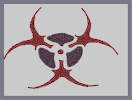Hover over the thumbnail for a full-size version.

Author im_bad_at_N author:im_bad_at_n biohazard ibannart rated zthing 2010-01-16 2010-07-25 3 by 37 people. \$NumaHazard#im_bad_at_N#biohazard#00000000000000000000000000000000000000000000000000000000000000000000000000000000000000000000000000000000000000000000000000000000000000000000000000000000000000000000000000000000000000000000000000000000000000000000000000000000000000000000000000000000000000000000000000000000000000000000000000000000000000000000000000000000000000000000000000000000000000000000000000000000000000000000000000000000000000000000000000000000000000000000000000000000000000000000000000000000000000000000000000000000000000000000000000000000000000000000000000000000000000000000000000000000000000000000000000000000000000000000000000000000000000000000000000000000000000000000000000000000000000000000000000000000000000000000000000000000000000000|12^360,312!12^366,312!12^372,312!12^366,306!12^360,306!12^354,312!12^354,312!12^354,306!12^372,306!12^354,318!12^360,318!12^366,318!12^372,318!12^378,312!12^366,300!12^372,319!12^375,317!12^377,314!12^378,310!12^377,307!12^375,305!12^373,303!12^370,301!12^366,301!12^355,303!12^359,300!12^364,299!12^351,317!12^349,313!12^349,310!12^350,306!12^351,318!12^353,319!12^355,321!12^359,321!12^363,322!12^368,322!12^372,320!12^504,240!12^492,240!12^498,240!12^486,240!12^480,240!12^474,240!12^468,240!12^480,234!12^474,234!12^474,234!12^468,234!12^486,234!12^498,234!12^504,234!12^492,234!12^492,228!12^480,228!12^480,228!12^468,228!12^474,228!12^480,228!12^480,234!12^486,228!12^480,252!12^480,252!12^486,246!12^492,252!12^492,252!12^498,258!12^498,252!12^504,252!12^504,252!12^504,258!12^504,258!12^504,264!12^504,246!12^492,246!12^498,246!12^486,252!12^492,258!12^486,258!12^480,258!12^468,258!12^474,258!12^462,258!12^456,258!12^468,252!12^474,252!12^474,246!12^480,246!12^468,246!12^462,252!12^444,264!12^450,264!12^438,270!12^432,270!12^420,276!12^426,276!12^432,276!12^426,270!12^426,282!12^420,270!12^444,270!12^510,264!12^510,258!12^510,252!12^516,246!12^510,246!12^510,246!12^510,240!12^510,234!12^510,228!12^510,228!12^510,222!12^510,216!12^510,210!12^510,204!12^510,198!12^504,210!12^504,216!12^504,222!12^504,228!12^504,228!12^498,228!12^498,222!12^498,216!12^498,210!12^492,222!12^492,222!12^486,222!12^480,222!12^474,222!12^468,222!12^423,284!12^426,282!12^428,282!12^430,280!12^433,279!12^434,278!12^437,276!12^439,274!12^442,273!12^444,271!12^446,270!12^448,269!12^450,268!12^453,267!12^455,265!12^457,265!12^460,264!12^422,283!12^421,282!12^419,281!12^417,279!12^414,276!12^413,273!12^419,273!12^416,271!12^422,269!12^424,269!12^425,269!12^429,266!12^434,265!12^437,264!12^439,263!12^442,261!12^444,261!12^447,259!12^450,258!12^455,255!12^457,253!12^460,251!12^464,249!12^467,222!12^466,221!12^463,245!12^466,243!12^467,239!12^451,212!12^454,213!12^458,217!12^461,219!12^465,222!12^463,219!12^470,219!12^474,219!12^480,219!12^484,217!12^486,217!12^489,216!12^491,214!12^495,212!12^499,210!12^502,207!12^504,206!12^507,204!12^507,202!12^508,199!12^509,197!12^511,193!12^499,59!12^501,61!12^503,64!12^506,66!12^508,68!12^510,72!12^513,77!12^515,80!12^517,84!12^518,88!12^531,117!12^530,123!12^528,120!12^528,120!12^528,132!12^528,132!12^528,126!12^534,120!12^534,126!12^534,132!12^534,138!12^534,138!12^534,144!12^534,150!12^534,156!12^534,162!12^534,168!12^534,174!12^528,174!12^528,168!12^528,168!12^528,162!12^528,180!12^528,186!12^528,192!12^528,204!12^528,210!12^528,198!12^522,198!12^522,204!12^522,210!12^516,210!12^516,204!12^516,198!12^516,192!12^522,186!12^522,192!12^522,180!12^540,144!12^540,150!12^540,156!12^540,168!12^540,162!12^540,138!12^534,114!12^528,108!12^534,186!12^534,180!12^540,174!12^540,180!12^534,216!12^540,222!12^534,228!12^534,210!12^534,204!12^534,198!12^534,198!12^534,198!12^534,192!12^534,228!12^534,222!12^522,222!12^522,222!12^516,222!12^522,222!12^528,216!12^522,222!12^516,216!12^522,216!12^528,222!12^516,222!12^516,228!12^516,228!12^522,228!12^528,228!12^522,234!12^522,234!12^516,240!12^522,240!12^522,240!12^528,240!12^528,240!12^528,234!12^534,234!12^534,240!12^534,240!12^540,240!12^546,234!12^546,234!12^540,234!12^540,234!12^540,228!12^516,234!12^522,252!12^522,252!12^522,246!12^534,246!12^534,246!12^540,246!12^540,246!12^528,246!12^528,252!12^516,252!12^516,258!12^546,246!12^546,240!12^552,240!12^552,246!12^558,246!12^558,240!12^570,246!12^570,246!12^564,246!12^564,240!12^570,240!12^552,234!12^558,234!12^576,240!12^576,246!12^582,246!12^576,240!12^582,240!12^582,246!12^594,246!12^588,240!12^588,246!12^600,252!12^606,246!12^606,252!12^600,246!12^588,252!12^588,252!12^594,252!12^534,252!12^612,252!12^618,252!12^624,258!12^624,252!12^636,258!12^630,258!12^642,264!12^636,264!12^648,270!12^654,270!12^660,276!12^514,190!12^515,186!12^516,184!12^518,182!12^520,179!12^520,176!12^521,174!12^522,171!12^523,168!12^525,166!12^526,164!12^526,161!12^526,158!12^528,154!12^529,150!12^529,147!12^529,145!12^529,142!12^529,138!12^520,91!12^521,92!12^523,96!12^524,98!12^526,102!12^526,104!12^527,108!12^528,111!12^528,117!12^528,122!12^518,86!12^519,88!12^520,92!12^524,97!12^526,101!12^527,103!12^530,108!12^531,112!12^534,118!12^535,123!12^536,126!12^537,129!12^538,132!12^539,137!12^539,141!12^539,144!12^540,149!12^537,184!12^542,169!12^542,166!12^542,162!12^543,158!12^543,156!12^542,154!12^542,154!12^542,152!12^543,148!12^544,143!12^544,140!12^542,137!12^541,133!12^539,128!12^539,128!12^539,128!12^538,127!12^537,123!12^536,118!12^536,116!12^536,189!12^545,228!12^547,228!12^550,229!12^553,231!12^555,232!12^559,233!12^562,234!12^565,235!12^568,234!12^573,236!12^577,237!12^583,238!12^583,238!12^587,239!12^591,240!12^592,240!12^595,240!12^597,241!12^601,243!12^607,245!12^609,246!12^612,247!12^615,247!12^617,248!12^627,252!12^630,255!12^630,255!12^632,256!12^635,257!12^639,258!12^641,260!12^644,262!12^647,264!12^651,266!12^653,268!12^657,271!12^660,275!12^661,276!12^666,279!12^669,282!12^672,284!12^674,286!12^678,289!12^678,289!12^681,292!12^684,296!12^688,301!12^691,305!12^695,311!12^697,313!12^700,317!12^703,321!12^705,325!12^597,254!12^601,256!12^605,257!12^606,258!12^612,260!12^616,261!12^618,262!12^619,262!12^624,263!12^629,265!12^635,266!12^636,267!12^638,269!12^643,270!12^647,272!12^650,273!12^657,275!12^661,279!12^667,282!12^609,258!12^616,258!12^620,258!12^555,251!12^560,251!12^565,252!12^568,252!12^578,252!12^579,252!12^575,252!12^567,252!12^579,253!12^586,251!12^533,252!12^540,252!12^546,252!12^550,252!12^512,267!12^514,264!12^518,260!12^524,256!12^528,254!12^510,278!12^511,274!12^510,268!12^509,275!12^508,272!12^505,269!12^503,267!12^499,265!12^464,265!12^467,264!12^476,263!12^480,263!12^482,263!12^485,263!12^489,263!12^492,263!12^494,264!12^468,263!12^354,438!12^360,438!12^366,438!12^384,438!12^372,438!12^378,438!12^408,438!12^396,432!12^390,438!12^396,438!12^402,438!12^402,432!12^408,432!12^414,426!12^414,432!12^414,438!12^408,426!12^402,426!12^390,420!12^384,426!12^390,426!12^396,426!12^390,432!12^384,432!12^378,432!12^372,432!12^372,426!12^372,426!12^372,414!12^372,408!12^372,402!12^372,390!12^372,390!12^372,384!12^372,372!12^372,372!12^372,384!12^372,378!12^372,402!12^372,396!12^372,420!12^378,426!12^378,420!12^378,414!12^378,408!12^378,402!12^384,414!12^384,420!12^366,432!12^366,426!12^366,426!12^366,420!12^366,414!12^366,408!12^366,402!12^366,396!12^366,396!12^366,390!12^366,384!12^366,378!12^366,372!12^366,366!12^360,366!12^372,366!12^354,426!12^360,426!12^360,432!12^354,426!12^360,420!12^360,414!12^354,420!12^354,426!12^348,432!12^348,432!12^348,432!12^354,432!12^342,426!12^342,426!12^348,426!12^336,426!12^330,426!12^324,426!12^330,432!12^336,432!12^342,432!12^336,438!12^342,438!12^342,438!12^348,438!12^330,438!12^330,444!12^330,450!12^336,450!12^342,444!12^366,450!12^372,450!12^354,450!12^342,450!12^348,450!12^360,450!12^360,444!12^372,444!12^360,444!12^354,444!12^348,444!12^336,444!12^366,444!12^312,486!12^318,486!12^318,480!12^324,480!12^330,474!12^330,474!12^330,468!12^336,468!12^336,468!12^336,462!12^336,462!12^342,462!12^342,456!12^336,456!12^330,456!12^330,462!12^324,462!12^324,468!12^324,474!12^318,474!12^312,480!12^324,486!12^330,480!12^336,474!12^348,468!12^348,462!12^348,456!12^342,468!12^300,498!12^300,492!12^300,492!12^306,486!12^300,486!12^306,492!12^288,498!12^294,492!12^294,498!12^288,504!12^282,504!12^276,510!12^276,510!12^270,510!12^276,504!12^258,516!12^264,516!12^234,522!12^252,516!12^170,525!12^174,525!12^177,525!12^178,525!12^180,526!12^182,526!12^185,527!12^185,527!12^189,527!12^193,527!12^195,528!12^202,529!12^206,529!12^214,528!12^192,527!12^195,528!12^199,528!12^206,528!12^210,527!12^215,526!12^219,526!12^221,526!12^228,526!12^230,526!12^239,523!12^246,521!12^231,521!12^236,521!12^242,520!12^247,520!12^251,518!12^254,517!12^258,516!12^265,515!12^269,514!12^274,512!12^277,510!12^283,508!12^287,507!12^291,504!12^295,502!12^300,500!12^304,498!12^310,495!12^318,491!12^321,489!12^326,486!12^328,483!12^334,480!12^338,477!12^343,474!12^347,471!12^349,468!12^353,463!12^310,493!12^311,494!12^315,491!12^310,489!12^217,523!12^224,522!12^229,521!12^234,519!12^237,519!12^240,518!12^243,516!12^245,516!12^247,515!12^251,513!12^256,512!12^261,510!12^263,509!12^266,506!12^270,504!12^274,502!12^277,500!12^280,498!12^284,495!12^287,494!12^292,491!12^292,491!12^296,488!12^298,487!12^299,485!12^301,484!12^304,480!12^305,479!12^310,476!12^314,475!12^315,473!12^308,475!12^313,472!12^314,470!12^317,468!12^320,465!12^321,464!12^323,462!12^326,460!12^329,456!12^329,456!12^331,453!12^312,423!12^315,423!12^317,424!12^321,427!12^323,429!12^326,431!12^329,434!12^320,422!12^324,422!12^324,423!12^329,423!12^332,423!12^335,422!12^340,422!12^340,422!12^345,420!12^346,418!12^349,417!12^353,415!12^355,412!12^360,408!12^360,407!12^360,404!12^359,369!12^360,371!12^361,374!12^362,379!12^361,381!12^360,385!12^360,391!12^360,394!12^360,398!12^356,363!12^356,362!12^360,362!12^362,363!12^365,364!12^368,364!12^369,364!12^374,365!12^376,365!12^377,365!12^378,365!12^377,368!12^376,372!12^374,378!12^374,381!12^375,386!12^376,392!12^377,392!12^375,383!12^375,378!12^376,371!12^378,401!12^378,399!12^376,397!12^375,393!12^377,373!12^376,377!12^376,381!12^376,384!12^376,389!12^377,394!12^378,399!12^379,402!12^381,405!12^381,406!12^381,406!12^381,406!12^382,412!12^385,412!12^388,413!12^392,415!12^394,417!12^396,418!12^394,422!12^398,422!12^400,421!12^403,421!12^407,423!12^410,423!12^413,423!12^418,423!12^420,422!12^421,422!12^423,422!12^420,423!12^396,456!12^390,456!12^396,450!12^390,450!12^384,450!12^378,450!12^378,444!12^390,444!12^396,444!12^402,444!12^384,444!12^408,444!12^414,444!12^414,450!12^414,456!12^408,456!12^402,450!12^408,456!12^408,450!12^408,456!12^402,456!12^384,456!12^396,462!12^402,462!12^408,462!12^414,462!12^414,462!12^420,462!12^420,456!12^426,468!12^420,468!12^414,468!12^402,468!12^408,468!12^414,474!12^420,474!12^426,474!12^426,474!12^432,474!12^414,480!12^420,480!12^426,480!12^432,480!12^438,480!12^438,480!12^438,480!12^444,480!12^438,474!12^408,474!12^426,486!12^432,486!12^438,486!12^444,486!12^444,486!12^450,486!12^444,492!12^450,492!12^450,492!12^456,498!12^456,492!12^462,492!12^468,498!12^462,498!12^474,498!12^474,504!12^480,504!12^486,504!12^486,504!12^492,510!12^498,510!12^498,510!12^504,510!12^516,510!12^510,510!12^356,460!12^352,462!12^352,459!12^357,457!12^361,456!12^360,460!12^360,461!12^363,459!12^366,458!12^366,458!12^367,458!12^373,458!12^377,458!12^378,459!12^379,459!12^385,459!12^389,460!12^391,462!12^395,464!12^397,467!12^400,469!12^403,471!12^406,472!12^409,475!12^413,478!12^417,480!12^420,483!12^425,485!12^427,485!12^431,488!12^434,489!12^435,490!12^438,492!12^442,494!12^444,495!12^446,496!12^450,498!12^454,500!12^458,502!12^462,503!12^466,504!12^471,506!12^472,506!12^476,506!12^480,507!12^485,509!12^485,509!12^487,510!12^489,511!12^494,512!12^508,515!12^495,513!12^500,514!12^504,515!12^517,517!12^518,517!12^513,515!12^516,516!12^520,516!12^529,516!12^531,516!12^536,516!12^538,516!12^540,516!12^542,515!12^546,515!12^548,514!12^549,514!12^553,514!12^556,514!12^562,514!12^567,513!12^572,512!12^574,512!12^581,512!12^584,511!12^588,510!12^525,519!12^519,511!12^523,513!12^528,515!12^533,515!12^525,510!12^522,512!12^530,514!12^534,515!12^462,490!12^466,492!12^469,494!12^473,496!12^477,498!12^481,499!12^484,500!12^488,502!12^492,503!12^497,505!12^501,506!12^507,508!12^510,508!12^425,461!12^427,463!12^430,466!12^433,469!12^435,470!12^438,473!12^441,475!12^445,478!12^447,480!12^448,480!12^448,480!12^452,483!12^453,484!12^455,487!12^457,488!12^418,455!12^415,452!12^414,449!12^413,443!12^415,433!12^416,428!12^216,258!12^216,252!12^216,246!12^216,240!12^216,234!12^216,228!12^216,216!12^216,210!12^216,222!12^216,222!12^222,204!12^222,210!12^222,216!12^222,222!12^222,228!12^222,234!12^222,234!12^222,240!12^222,246!12^222,252!12^222,258!12^222,264!12^216,270!12^216,264!12^222,270!12^228,264!12^228,264!12^228,258!12^228,252!12^228,252!12^228,246!12^228,240!12^228,234!12^228,228!12^228,222!12^234,216!12^228,210!12^228,216!12^234,222!12^234,234!12^234,240!12^234,246!12^234,246!12^234,258!12^234,258!12^234,258!12^234,252!12^234,264!12^234,228!12^240,222!12^240,222!12^240,228!12^240,240!12^240,240!12^240,252!12^240,252!12^240,264!12^246,252!12^240,258!12^240,246!12^240,234!12^246,222!12^246,222!12^246,234!12^246,240!12^246,246!12^246,228!12^258,222!12^252,228!12^252,234!12^258,240!12^258,252!12^258,252!12^252,252!12^252,246!12^252,240!12^258,246!12^258,228!12^258,222!12^258,228!12^258,234!12^252,228!12^252,222!12^246,264!12^252,264!12^258,264!12^264,264!12^270,264!12^270,264!12^276,270!12^246,258!12^252,258!12^258,258!12^264,258!12^264,258!12^270,258!12^270,258!12^276,264!12^282,264!12^288,270!12^282,270!12^294,270!12^300,276!12^300,270!12^306,276!12^294,276!12^300,282!12^306,276!12^312,276!12^210,264!12^204,258!12^204,258!12^186,258!12^180,264!12^144,264!12^150,264!12^162,264!12^168,264!12^174,264!12^156,264!12^156,258!12^162,258!12^168,258!12^174,258!12^174,258!12^180,258!12^198,258!12^192,258!12^210,258!12^210,258!12^210,252!12^204,252!12^198,252!12^192,252!12^186,252!12^180,252!12^180,252!12^168,252!12^168,252!12^174,246!12^180,252!12^174,252!12^180,246!12^192,246!12^198,246!12^198,246!12^204,246!12^210,246!12^180,246!12^186,246!12^126,258!12^132,258!12^132,258!12^138,258!12^144,258!12^144,258!12^150,258!12^138,252!12^144,252!12^144,252!12^150,252!12^156,252!12^162,252!12^156,246!12^162,246!12^168,246!12^186,240!12^192,240!12^198,240!12^204,240!12^210,240!12^198,234!12^204,234!12^210,234!12^210,234!12^192,264!12^192,264!12^198,264!12^204,264!12^210,270!12^216,276!12^114,264!12^120,264!12^120,264!12^126,264!12^126,264!12^138,264!12^132,264!12^120,270!12^114,270!12^114,270!12^102,270!12^96,270!12^108,270!12^108,270!12^120,258!12^108,264!12^84,276!12^90,276!12^96,276!12^102,276!12^84,282!12^78,282!12^90,282!12^72,288!12^72,288!12^78,288!12^66,294!12^60,300!12^54,306!12^204,228!12^210,228!12^26,353!12^26,347!12^27,346!12^30,343!12^31,341!12^32,339!12^32,338!12^33,338!12^34,336!12^35,333!12^36,331!12^38,327!12^42,322!12^45,316!12^47,314!12^47,318!12^47,316!12^50,315!12^53,313!12^57,311!12^61,306!12^62,304!12^65,301!12^69,298!12^70,298!12^77,295!12^77,290!12^80,290!12^84,289!12^49,311!12^52,309!12^55,306!12^55,305!12^56,302!12^57,298!12^60,296!12^62,294!12^64,292!12^69,289!12^73,287!12^76,284!12^79,282!12^90,274!12^94,271!12^96,269!12^99,267!12^104,265!12^70,294!12^76,293!12^89,283!12^91,285!12^91,285!12^95,283!12^98,280!12^100,279!12^104,279!12^106,278!12^109,276!12^114,273!12^116,273!12^122,271!12^123,271!12^128,269!12^131,268!12^135,268!12^138,268!12^142,267!12^145,267!12^151,266!12^75,282!12^78,278!12^113,261!12^115,258!12^122,258!12^125,257!12^127,256!12^127,255!12^130,252!12^134,252!12^139,250!12^141,249!12^145,248!12^149,247!12^151,246!12^152,246!12^158,246!12^161,246!12^163,244!12^165,243!12^169,243!12^174,242!12^178,241!12^181,240!12^185,239!12^187,238!12^190,236!12^192,235!12^194,233!12^212,298!12^213,297!12^213,293!12^216,286!12^214,287!12^214,283!12^213,278!12^212,276!12^207,272!12^186,264!12^191,266!12^194,266!12^198,267!12^201,269!12^204,270!12^218,278!12^220,276!12^224,272!12^228,268!12^232,266!12^229,269!12^235,269!12^240,267!12^243,267!12^250,268!12^254,268!12^261,267!12^266,268!12^270,269!12^274,271!12^279,273!12^282,273!12^286,276!12^288,279!12^291,281!12^295,283!12^299,285!12^301,287!12^302,288!12^301,284!12^318,272!12^312,276!12^310,278!12^308,280!12^306,282!12^304,284!12^286,263!12^288,265!12^293,268!12^296,270!12^301,272!12^304,272!12^307,273!12^311,274!12^309,272!12^261,242!12^262,245!12^263,248!12^265,251!12^266,252!12^267,253!12^272,256!12^274,256!12^277,259!12^279,261!12^272,215!12^269,216!12^265,217!12^263,218!12^262,219!12^260,222!12^259,225!12^259,229!12^260,233!12^260,240!12^226,205!12^228,206!12^231,209!12^236,213!12^239,215!12^243,217!12^247,218!12^252,219!12^255,220!12^258,218!12^261,218!12^198,144!12^198,150!12^198,156!12^198,156!12^198,162!12^198,168!12^198,174!12^198,174!12^198,180!12^198,180!12^198,186!12^198,192!12^198,198!12^198,204!12^198,210!12^204,216!12^204,210!12^204,204!12^204,198!12^210,192!12^210,186!12^204,180!12^204,174!12^204,168!12^204,168!12^204,162!12^204,192!12^204,186!12^210,204!12^210,210!12^210,216!12^204,222!12^204,222!12^210,222!12^210,198!12^216,198!12^216,204!12^216,192!12^210,186!12^192,180!12^192,174!12^192,168!12^192,162!12^192,162!12^192,150!12^192,150!12^192,138!12^192,132!12^192,144!12^192,156!12^186,144!12^186,150!12^186,156!12^186,162!12^198,138!12^198,132!12^198,126!12^198,120!12^198,114!12^204,108!12^204,102!12^204,96!12^204,114!12^192,126!12^204,156!12^198,230!12^200,227!12^200,224!12^200,221!12^200,219!12^200,216!12^186,170!12^187,172!12^187,175!12^188,180!12^188,181!12^190,183!12^191,186!12^192,189!12^194,194!12^195,196!12^199,202!12^209,168!12^209,169!12^209,175!12^211,179!12^214,182!12^217,186!12^219,192!12^222,197!12^225,199!12^228,205!12^222,200!12^218,196!12^216,192!12^213,185!12^209,177!12^184,151!12^183,146!12^187,139!12^185,137!12^185,135!12^186,131!12^190,125!12^191,122!12^192,119!12^192,117!12^195,113!12^195,111!12^199,107!12^204,102!12^197,107!12^201,103!12^204,97!12^206,93!12^207,90!12^209,87!12^210,87!12^210,86!12^213,81!12^214,79!12^217,77!12^218,75!12^219,73!12^221,70!12^224,68!12^226,65!12^228,63!12^232,58!12^237,54!12^238,53!12^242,49!12^246,46!12^205,153!12^203,150!12^203,147!12^203,145!12^203,141!12^203,137!12^203,132!12^203,129!12^203,125!12^204,121!12^204,115!12^206,109!12^207,105!12^208,101!12^184,167!12^182,163!12^182,155!10^290,233!10^294,229!10^296,228!10^300,227!10^303,225!10^305,224!10^306,224!10^311,222!10^313,220!10^315,220!10^318,220!10^322,219!10^323,219!10^327,217!10^329,216!10^330,216!10^335,215!10^337,215!10^340,214!10^341,214!10^344,214!10^349,212!10^352,212!10^356,212!10^356,212!10^361,212!10^366,212!10^369,212!10^362,210!10^367,210!10^374,210!10^375,210!10^378,210!10^381,211!10^388,212!10^389,212!10^391,213!10^393,214!10^396,215!10^400,217!10^402,217!10^406,219!10^410,220!10^412,221!10^412,221!10^414,221!10^419,223!10^423,224!10^427,226!10^430,227!10^433,228!10^435,230!10^437,231!10^438,231!10^374,272!10^374,270!10^375,267!10^376,265!10^376,263!10^379,262!10^382,259!10^384,257!10^385,256!10^390,254!10^392,254!10^395,254!10^397,253!10^402,251!10^404,250!10^408,249!10^411,249!10^414,247!10^416,247!10^418,246!10^423,245!10^423,245!10^425,244!10^426,243!10^430,242!10^432,240!10^434,239!10^436,235!10^437,233!10^437,233!10^290,233!10^295,235!10^296,236!10^300,237!10^302,238!10^305,240!10^308,241!10^311,242!10^311,242!10^315,245!10^319,246!10^320,246!10^322,247!10^326,248!10^331,249!10^334,250!10^334,250!10^336,250!10^340,251!10^341,251!10^345,254!10^348,255!10^353,257!10^356,259!10^358,262!10^360,264!10^363,267!10^364,269!10^365,270!10^368,272!10^370,272!10^371,271!10^368,268!10^366,264!10^366,261!10^363,259!10^358,256!10^353,253!10^348,251!10^370,263!10^368,262!10^364,256!10^360,253!10^357,250!10^355,247!10^355,240!10^355,236!10^355,228!10^355,220!10^355,220!10^355,220!10^355,226!10^355,230!10^355,219!10^353,216!10^306,229!10^308,229!10^315,227!10^320,225!10^321,224!10^325,223!10^329,223!10^335,221!10^339,220!10^340,219!10^341,219!10^347,219!10^351,218!10^331,221!10^320,227!10^313,228!10^307,231!10^304,234!10^311,235!10^317,234!10^319,233!10^324,230!10^312,233!10^309,233!10^307,234!10^304,233!10^311,230!10^316,230!10^321,229!10^324,228!10^332,226!10^296,233!10^304,232!10^309,230!10^328,225!10^328,225!10^299,234!10^313,238!10^316,240!10^319,240!10^322,239!10^325,236!10^326,235!10^317,238!10^324,243!10^329,243!10^335,243!10^337,244!10^342,247!10^347,247!10^350,247!10^331,245!10^328,240!10^334,239!10^338,240!10^343,242!10^346,243!10^350,242!10^336,245!10^336,235!10^331,232!10^328,229!10^335,226!10^339,223!10^334,229!10^334,230!10^336,234!10^343,231!10^343,226!10^348,220!10^343,225!10^339,230!10^333,234!10^332,236!10^342,235!10^343,235!10^347,237!10^350,232!10^348,227!10^345,223!10^345,221!10^349,218!10^351,217!10^351,216!10^349,225!10^350,236!10^351,230!10^351,229!10^346,235!10^359,218!10^359,218!10^359,224!10^360,231!10^360,236!10^360,242!10^360,244!10^360,231!10^360,223!10^363,219!10^364,222!10^362,233!10^361,239!10^362,249!10^362,239!10^362,229!10^362,225!10^364,225!10^368,221!10^369,217!10^370,221!10^368,229!10^368,236!10^367,241!10^367,247!10^367,254!10^368,259!10^368,250!10^368,236!10^370,228!10^369,224!10^365,220!10^365,217!10^373,218!10^373,217!10^374,216!10^376,219!10^372,227!10^372,232!10^373,238!10^373,246!10^373,252!10^372,257!10^372,261!10^370,249!10^370,231!10^381,219!10^383,215!10^384,220!10^383,225!10^382,229!10^381,234!10^381,239!10^379,248!10^379,256!10^379,260!10^379,251!10^379,241!10^378,234!10^378,227!10^379,221!10^380,237!10^378,251!10^376,256!10^378,232!10^376,225!10^375,224!10^387,221!10^389,220!10^391,222!10^394,223!10^400,224!10^401,225!10^401,225!10^407,225!10^411,226!10^416,227!10^421,228!10^425,229!10^430,229!10^434,231!10^397,220!10^404,221!10^407,222!10^391,224!10^398,226!10^404,228!10^408,229!10^414,231!10^419,231!10^425,234!10^391,227!10^398,230!10^403,231!10^409,233!10^413,234!10^416,236!10^423,238!10^422,231!10^421,230!10^418,233!10^383,230!10^393,229!10^395,229!10^398,227!10^402,233!10^407,235!10^412,236!10^415,238!10^419,239!10^421,239!10^425,235!10^433,236!10^424,236!10^422,236!10^415,241!10^410,243!10^406,244!10^399,247!10^395,247!10^391,248!10^388,249!10^384,248!10^390,241!10^388,237!10^387,233!10^387,229!10^391,231!10^393,237!10^397,240!10^400,241!10^398,236!10^398,236!10^402,238!10^405,240!10^386,250!10^386,254!10^390,243!10^393,236!10^396,231!10^395,230!10^387,236!10^384,243!10^379,254!10^376,262!10^397,244!10^400,244!10^404,245!10^410,245!10^413,245!10^422,241!10^408,239!10^391,241!10^384,214!10^384,212!10^237,296!10^236,298!10^235,305!10^235,308!10^235,312!10^236,318!10^237,321!10^238,327!10^239,330!10^240,335!10^241,339!10^242,342!10^245,347!10^248,352!10^251,354!10^252,355!10^254,358!10^255,359!10^257,363!10^258,364!10^261,367!10^262,369!10^266,371!10^267,373!10^269,376!10^271,378!10^273,380!10^275,383!10^278,385!10^281,387!10^285,388!10^287,390!10^290,392!10^293,393!10^295,395!10^299,397!10^306,400!10^308,401!10^310,402!10^315,405!10^317,405!10^319,406!10^298,399!10^303,401!10^305,402!10^294,348!10^288,348!10^282,348!10^276,348!10^276,348!10^270,348!10^264,348!10^264,348!10^258,348!10^258,342!10^258,342!10^264,342!10^270,348!10^270,342!10^276,342!10^282,342!10^288,342!10^288,342!10^294,342!10^300,342!10^306,342!10^312,342!10^312,342!10^306,348!10^306,348!10^300,348!10^312,348!10^312,354!10^312,360!10^312,366!10^312,366!10^312,378!10^312,378!10^312,396!10^312,402!10^312,390!10^312,390!10^312,384!10^312,372!10^306,354!10^306,360!10^306,366!10^306,372!10^306,378!10^306,384!10^306,390!10^306,390!10^306,396!10^300,390!10^300,384!10^300,378!10^300,372!10^300,372!10^300,360!10^300,360!10^300,354!10^294,354!10^294,360!10^294,366!10^294,378!10^294,390!10^294,378!10^294,372!10^294,378!10^294,384!10^288,384!10^288,378!10^288,378!10^288,366!10^288,366!10^288,360!10^288,354!10^288,372!10^282,354!10^282,360!10^282,366!10^282,372!10^282,378!10^288,384!10^276,372!10^276,366!10^276,360!10^276,360!10^270,354!10^270,360!10^270,366!10^270,372!10^264,366!10^264,360!10^264,354!10^264,354!10^258,354!10^264,360!10^300,366!10^276,354!10^276,378!10^288,384!10^252,342!10^252,336!10^252,336!10^252,330!10^252,324!10^252,318!10^252,306!10^252,306!10^252,294!10^252,312!10^252,300!10^246,300!10^246,306!10^246,318!10^246,324!10^240,324!10^246,330!10^246,312!10^258,300!10^258,300!10^258,306!10^258,312!10^258,318!10^258,324!10^258,330!10^258,330!10^258,330!10^270,330!10^270,330!10^282,330!10^294,330!10^300,330!10^306,330!10^306,330!10^312,330!10^306,330!10^306,336!10^300,336!10^294,336!10^294,336!10^288,336!10^282,336!10^276,336!10^270,336!10^270,336!10^264,336!10^258,336!10^264,330!10^276,330!10^288,330!10^288,318!10^282,318!10^276,324!10^270,324!10^270,318!10^270,318!10^276,318!10^276,318!10^270,324!10^270,324!10^264,324!10^264,318!10^264,318!10^264,312!10^264,306!10^264,306!10^276,312!10^276,306!10^270,306!10^270,312!10^282,312!10^288,324!10^282,324!10^294,318!10^294,318!10^294,324!10^294,324!10^300,324!10^306,324!10^312,324!10^245,337!10^250,334!10^250,329!10^249,322!10^250,316!10^250,309!10^250,304!10^255,304!10^256,311!10^254,317!10^254,323!10^254,330!10^254,338!10^255,344!10^256,354!10^258,335!10^255,329!10^251,344!10^249,347!10^246,344!10^246,338!10^245,335!10^244,333!10^242,327!10^242,323!10^242,320!10^242,315!10^241,311!10^241,305!10^241,302!10^243,297!10^240,307!10^240,312!10^239,315!10^239,316!10^239,306!10^240,302!10^296,386!10^295,382!10^297,375!10^295,371!10^296,366!10^298,361!10^297,357!10^296,353!10^297,349!10^298,346!10^297,341!10^297,338!10^297,333!10^297,330!10^297,326!10^289,324!10^292,337!10^292,341!10^290,347!10^290,351!10^290,359!10^290,365!10^288,374!10^289,381!10^289,385!10^288,387!10^289,376!10^290,371!10^291,369!10^284,382!10^279,382!10^284,373!10^278,376!10^273,375!10^281,370!10^286,368!10^273,369!10^277,369!10^283,364!10^277,364!10^272,364!10^267,364!10^262,364!10^265,358!10^272,357!10^277,357!10^281,358!10^285,353!10^278,353!10^272,354!10^268,354!10^262,354!10^261,359!10^262,352!10^273,351!10^279,351!10^289,353!10^285,359!10^274,360!10^269,369!10^251,350!10^259,350!10^270,352!10^265,344!10^270,344!10^275,344!10^281,344!10^263,335!10^270,336!10^274,337!10^278,335!10^285,335!10^291,334!10^274,330!10^262,332!10^258,332!10^266,334!10^268,334!10^275,334!10^258,333!10^263,339!10^257,347!10^255,354!10^263,348!10^255,340!10^254,333!10^253,348!10^265,352!10^284,346!10^283,340!10^277,340!10^261,345!10^264,328!10^264,328!10^271,329!10^272,329!10^263,326!10^259,323!10^265,322!10^269,322!10^273,323!10^262,317!10^266,317!10^270,317!10^273,317!10^274,317!10^284,314!10^240,293!10^242,291!10^245,291!10^250,291!10^256,293!10^258,293!10^262,295!10^265,297!10^267,299!10^271,300!10^275,301!10^279,303!10^281,304!10^283,306!10^287,309!10^290,311!10^237,300!10^243,298!10^248,296!10^253,296!10^256,296!10^259,299!10^265,302!10^270,305!10^274,308!10^276,310!10^267,309!10^263,309!10^261,308!10^260,306!10^262,304!10^264,303!10^264,301!10^242,307!10^247,325!10^257,322!10^283,321!10^286,321!10^287,321!10^293,321!10^296,320!10^297,320!10^300,320!10^306,320!10^311,320!10^313,320!10^313,320!10^305,318!10^298,318!10^294,318!10^291,316!10^291,315!10^292,313!10^297,315!10^316,321!10^320,321!10^322,322!10^323,325!10^321,327!10^321,330!10^320,331!10^318,334!10^316,336!10^315,338!10^314,341!10^304,326!10^308,329!10^321,340!10^318,342!10^318,346!10^318,350!10^318,352!10^318,356!10^319,362!10^321,365!10^322,368!10^325,376!10^326,378!10^326,384!10^323,405!10^326,403!10^327,399!10^328,396!10^328,392!10^326,390!10^320,399!10^321,397!10^325,393!10^325,391!10^324,389!10^323,385!10^322,381!10^322,377!10^320,374!10^318,368!10^311,341!10^312,351!10^312,356!10^315,367!10^315,372!10^316,378!10^316,383!10^317,391!10^318,394!10^316,395!10^315,396!10^315,390!10^315,387!10^318,383!10^319,389!10^313,398!10^305,396!10^302,395!10^299,393!10^299,390!10^305,391!10^309,394!10^309,387!10^303,385!10^303,384!10^306,382!10^307,381!10^306,377!10^301,375!10^301,374!10^308,374!10^309,374!10^305,371!10^304,369!10^308,367!10^310,364!10^307,363!10^304,363!10^303,362!10^304,360!10^308,358!10^306,355!10^305,354!10^305,354!10^308,349!10^309,349!10^307,346!10^305,344!10^305,340!10^305,336!10^305,334!10^288,328!10^285,328!10^281,327!10^280,322!10^280,321!10^311,337!10^311,334!10^313,332!10^316,351!10^314,360!10^315,364!10^316,366!10^319,362!10^402,328!10^404,332!10^405,333!10^405,339!10^406,345!10^406,348!10^407,352!10^406,355!10^405,359!10^406,364!10^406,368!10^407,372!10^408,375!10^408,378!10^408,382!10^408,385!10^408,388!10^409,392!10^412,395!10^413,396!10^414,397!10^418,396!10^419,395!10^422,395!10^425,393!10^426,391!10^428,391!10^433,390!10^431,391!10^436,387!10^439,387!10^443,386!10^443,385!10^444,384!10^447,382!10^451,379!10^452,378!10^454,376!10^457,374!10^458,372!10^459,371!10^462,370!10^464,368!10^466,364!10^467,363!10^468,360!10^471,356!10^472,355!10^474,352!10^475,351!10^478,348!10^478,346!10^480,343!10^481,339!10^482,336!10^484,335!10^485,333!10^486,330!10^488,326!10^488,324!10^489,320!10^490,318!10^490,312!10^491,309!10^491,306!10^491,303!10^491,298!10^490,295!10^489,292!10^488,289!10^486,286!10^485,284!10^432,315!10^434,312!10^437,309!10^438,307!10^443,305!10^446,301!10^449,299!10^451,298!10^454,295!10^455,294!10^460,291!10^403,329!10^403,326!10^403,323!10^405,320!10^407,319!10^411,319!10^416,318!10^419,318!10^422,317!10^423,316!10^428,314!10^431,313!10^434,310!10^465,286!10^468,284!10^476,284!10^477,284!10^479,284!10^474,284!10^469,285!10^436,311!10^414,324!10^412,324!10^410,325!10^412,327!10^421,321!10^425,321!10^427,320!10^416,323!10^420,321!10^426,320!10^433,318!10^437,315!10^440,312!10^441,311!10^444,309!10^446,307!10^451,303!10^453,300!10^455,299!10^456,298!10^459,295!10^462,294!10^466,292!10^468,291!10^470,291!10^471,291!10^476,290!10^480,290!10^481,290!10^484,295!10^483,293!10^487,300!10^484,305!10^482,310!10^481,316!10^479,320!10^478,324!10^475,328!10^472,333!10^469,341!10^465,349!10^458,357!10^462,352!10^466,349!10^471,345!10^472,341!10^473,338!10^475,334!10^477,330!10^479,329!10^481,325!10^482,321!10^484,317!10^485,313!10^487,307!10^488,302!10^485,295!10^484,289!10^490,313!10^487,318!10^483,326!10^482,327!10^474,340!10^473,343!10^470,349!10^465,354!10^463,358!10^460,363!10^455,372!10^453,374!10^457,368!10^462,361!10^465,355!10^468,352!10^470,349!10^473,346!10^480,339!10^481,337!10^481,335!10^424,387!10^430,385!10^433,382!10^438,379!10^440,377!10^442,376!10^446,373!10^448,371!10^453,367!10^455,365!10^457,360!10^429,385!10^438,380!10^442,379!10^449,375!10^414,389!10^418,388!10^423,388!10^429,386!10^437,382!10^441,379!10^414,389!10^415,389!10^416,381!10^416,379!10^415,375!10^414,370!10^413,364!10^412,359!10^412,355!10^411,351!10^411,344!10^411,336!10^409,331!10^410,338!10^411,342!10^412,356!10^411,360!10^410,365!10^410,370!10^410,374!10^410,378!10^412,383!10^414,330!10^417,328!10^423,325!10^427,324!10^441,321!10^430,325!10^434,323!10^439,322!10^447,317!10^449,316!10^451,313!10^454,308!10^456,306!10^463,302!10^464,300!10^466,299!10^471,297!10^474,297!10^479,297!10^431,321!10^439,317!10^441,315!10^447,311!10^451,308!10^453,305!10^460,301!10^463,298!10^480,294!10^472,295!10^479,293!10^459,310!10^454,313!10^458,312!10^463,308!10^470,306!10^473,304!10^476,304!10^477,304!10^459,305!10^468,305!10^470,304!10^474,303!10^481,303!10^481,305!10^415,333!10^416,335!10^415,339!10^414,344!10^414,348!10^414,352!10^415,356!10^415,360!10^416,366!10^418,371!10^418,373!10^419,377!10^421,380!10^419,383!10^415,387!10^416,391!10^421,334!10^421,334!10^420,336!10^419,342!10^419,346!10^420,351!10^420,355!10^420,358!10^421,364!10^422,369!10^424,374!10^426,377!10^427,378!10^418,365!10^419,332!10^425,330!10^426,333!10^426,340!10^425,344!10^424,350!10^424,354!10^424,359!10^424,365!10^427,370!10^430,374!10^423,378!10^423,378!10^428,379!10^430,379!10^408,336!10^433,330!10^431,334!10^431,337!10^431,340!10^430,343!10^429,345!10^429,349!10^429,353!10^429,357!10^429,361!10^430,365!10^433,369!10^435,371!10^436,371!10^422,383!10^418,329!10^423,341!10^436,328!10^441,326!10^445,324!10^447,323!10^451,321!10^453,320!10^456,318!10^460,315!10^463,314!10^467,314!10^468,313!10^471,311!10^475,310!10^472,314!10^471,319!10^471,321!10^467,326!10^466,330!10^466,331!10^465,334!10^463,337!10^461,339!10^460,343!10^458,346!10^456,349!10^454,353!10^450,357!10^447,363!10^442,367!10^439,369!10^445,364!10^450,362!10^453,361!10^457,355!10^458,350!10^460,346!10^464,341!10^466,335!10^470,330!10^473,324!10^476,319!10^479,312!10^479,303!10^481,298!10^473,327!10^463,338!10^462,342!10^468,340!10^465,343!10^460,350!10^459,354!10^456,358!10^453,363!10^449,367!10^444,372!10^442,374!10^439,365!10^435,364!10^434,364!10^434,361!10^436,357!10^436,354!10^436,350!10^436,346!10^436,343!10^436,341!10^437,337!10^439,334!10^440,334!10^443,334!10^443,337!10^442,339!10^442,342!10^442,346!10^442,348!10^441,351!10^441,354!10^441,357!10^439,360!10^443,360!10^446,358!10^446,357!10^446,353!10^448,348!10^448,344!10^448,340!10^448,337!10^448,333!10^448,330!10^449,326!10^453,326!10^454,330!10^452,333!10^452,339!10^452,344!10^451,348!10^450,351!10^452,348!10^455,340!10^455,335!10^456,329!10^458,323!10^462,326!10^459,333!10^457,336!10^458,331!10^459,325!10^462,322!10^465,320!10^466,319!10^445,329!10^441,332!10^436,338!10^432,347!10^431,354!10^431,359!10^425,341!10^428,335!10^432,329!10^425,327!10^406,342!10^427,349!10^441,351!10^442,352!10^450,367!10^436,369!10^434,369!10^433,372!10^447,368!10^475,335!10^465,330!10^474,315!10^463,315!10^459,319!10^454,316!10^476,298!10^475,286!10^289,226!10^287,228!10^287,226!10^290,224!10^294,222!10^297,221!10^299,221!10^302,220!10^305,219!10^308,217!10^312,215!10^313,214!10^315,213!10^318,212!10^323,211!10^324,211!10^326,210!10^329,210!10^333,209!10^336,207!10^338,207!10^342,207!10^343,206!10^345,206!10^347,206!10^351,205!10^353,205!10^356,205!10^358,205!10^361,205!10^364,204!10^365,204!10^296,224!10^305,223!10^318,219!10^312,219!10^316,217!10^322,215!10^331,213!10^335,212!10^340,210!10^340,210!10^348,208!10^351,208!10^353,208!10^357,207!10^327,214!10^369,203!10^373,203!10^377,203!10^380,204!10^382,204!10^385,204!10^386,204!10^386,204!10^391,206!10^393,208!10^397,208!10^399,208!10^403,211!10^406,212!10^409,213!10^411,213!10^412,213!10^416,215!10^418,215!10^421,216!10^424,218!10^427,219!10^430,220!10^433,222!10^435,223!10^436,224!10^438,226!10^440,227!10^370,207!10^374,208!10^376,208!10^379,208!10^385,209!10^386,210!10^393,214!10^395,215!10^398,215!10^402,215!10^405,216!10^408,217!10^412,218!10^413,218!10^418,220!10^420,221!10^392,211!10^385,210!10^390,224!10^391,225!10^396,212!10^386,205!10^385,205!10^387,217!10^386,222!10^386,226!10^338,232!10^355,244!10^350,237!10^357,237!10^365,235!10^373,235!10^376,241!10^376,242!10^381,248!10^382,248!10^377,250!10^377,250!10^388,245!10^393,245!10^370,242!12^492,216!12^492,217!12^479,222!12^472,263!12^427,419!12^353,455!12^364,455!12^370,454!12^376,454!12^348,421!12^282,500!12^525,106!12^525,110!12^527,116!12^496,56!12^493,52!12^492,50# Dedication to Zthing because of the recent mass sniping he received thanks to pakwan and his multi accounting. I feel your pain dude. Another n-art that i promised a while ago & finally getting too. Didnt spend a lot of time on it, since I am also subject to that guys sniping. Please rate my last n-art too, that got hit hard =(. Will redo this eventually Zthing, and make it a lot better. Edit: Tags

## Other maps by this author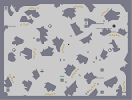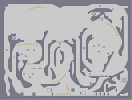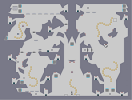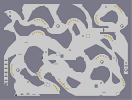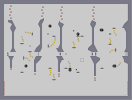Mining Ore Mining Copper Cave of Uncertainty Mining Iron They're not bunnies Revenge of the Baby-Sat

Pages: (0)

### Some guy stole your map.

http://www.nmaps.net/194380

### Thanks!

Except if I just read sniperassassin's comment I would've known it, lol.

### Thanks...

... with the knowledge of that definition on ecould deduce a "reverse sniping" is possible as well. There may already be a name for it, but I wouldn't know.

### I don't know what "sniping" is...

... But I like the look of this, even though it doesn't perticularly like Zthing's avatar.

### got it up to a 3 ^^

.

i would rate this a 5/5 regardless of antisniping.

:/

### I wasnt going to spend a whole lot of time

if the result would be the same... 1/5 or 2/5 thanks to that one guy. I wasnt feelin' the usual patience I have with n-arts after what happened to the hobbes one. sorry =(

### hmm

it sounds in the description like you spent less time on this because you were being sniped. that doesn't make sense to me.

### Holy shit dude

Your last two maps got seriously sniped

### 5/5 of course

Thanks a ton. I look forward to the redo, because I know what you're capable of. This means a lot.

### Neeeeaat

but a bit too far to the left.
4.5/5

### you got sniped

horribly on this.
5d to offset.

### ill check out that object mover

that might come in handy ;D

But im not disabling my ratings. Thats just giving him what he wants and doing so tells him he is "correct" when mass sniping is, by far, not proper social etiquette.
1. Why not use the object-mover [metanet.2.forumer.com]?
2. Why not disable ratings?

... Pretty well-made, by the way^^

### all i can say is

I'm looking forward to the re-do

### yeah, lol

i didnt bother to spend time centering it. =/

### I got sniped too

but he got sniped the worst. Love how it's off center XD